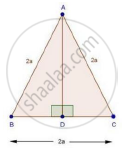Share

Books Shortlist
Your shortlist is empty

# Solution for In a Triangle Abc, Ab = Bc = Ca = 2a and Ad ⊥ Bc. Prove that - CBSE Class 10 - Mathematics

ConceptApplication of Pythagoras Theorem in Acute Angle and Obtuse Angle

#### Question

In a ΔABC, AB = BC = CA = 2a and AD ⊥ BC. Prove that

(i) AD = asqrt3

(ii) Area (ΔABC) = sqrt3 a2

#### Solution(i) In ΔABD and ΔACD

AB = AC                                  [Given]

Then, ΔABD ≅ ΔACD                 [By RHS condition]

∴ BD = CD = a                         [By c.p.c.t]

In ΔADB, by Pythagoras theorem

AD2 + BD2 = AB2

⇒ AD2 + (a)2 = (2a)2

⇒ AD2 + a2 = 4a2

⇒ AD2 = 4a2 − a2 = 3a2

⇒ AD = asqrt3

(ii) Area of ΔABC = 1/2xxBCxxAD

=1/2xx2axxasqrt3

=sqrt3 a2

Is there an error in this question or solution?

#### Video TutorialsVIEW ALL 

Solution for question: In a Triangle Abc, Ab = Bc = Ca = 2a and Ad ⊥ Bc. Prove that concept: Application of Pythagoras Theorem in Acute Angle and Obtuse Angle. For the course CBSE
S